Chapter 4 Part 1 - AD,AS and Related Concepts

Economics Class 12
Macroeconomics

## What is MPS?

MPS means Marginal Propensity to Save

It is ratio of Change in Saving to Change in Income

MPS = Change in Savings /Change in Income

= Delta S /Delta Y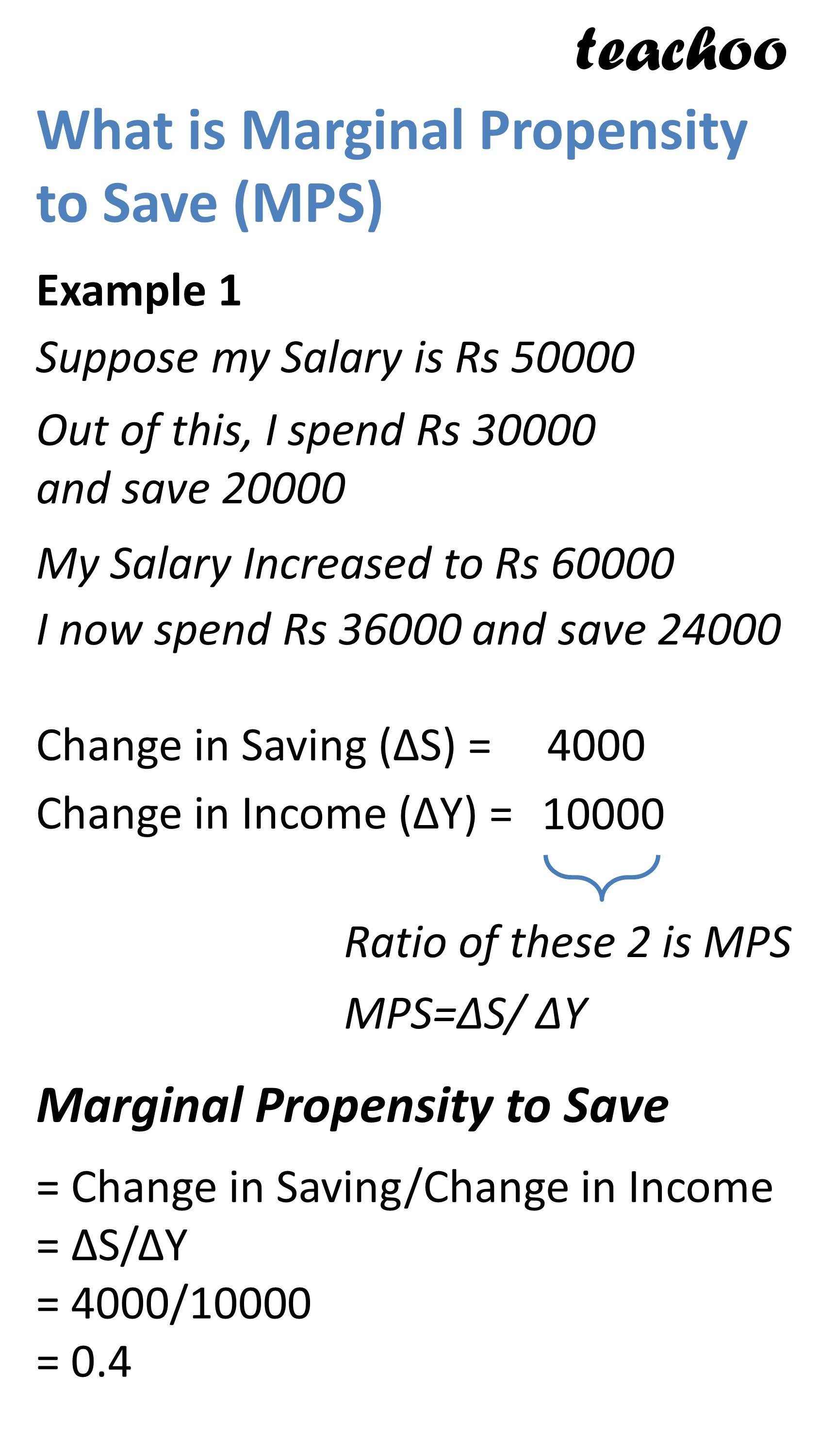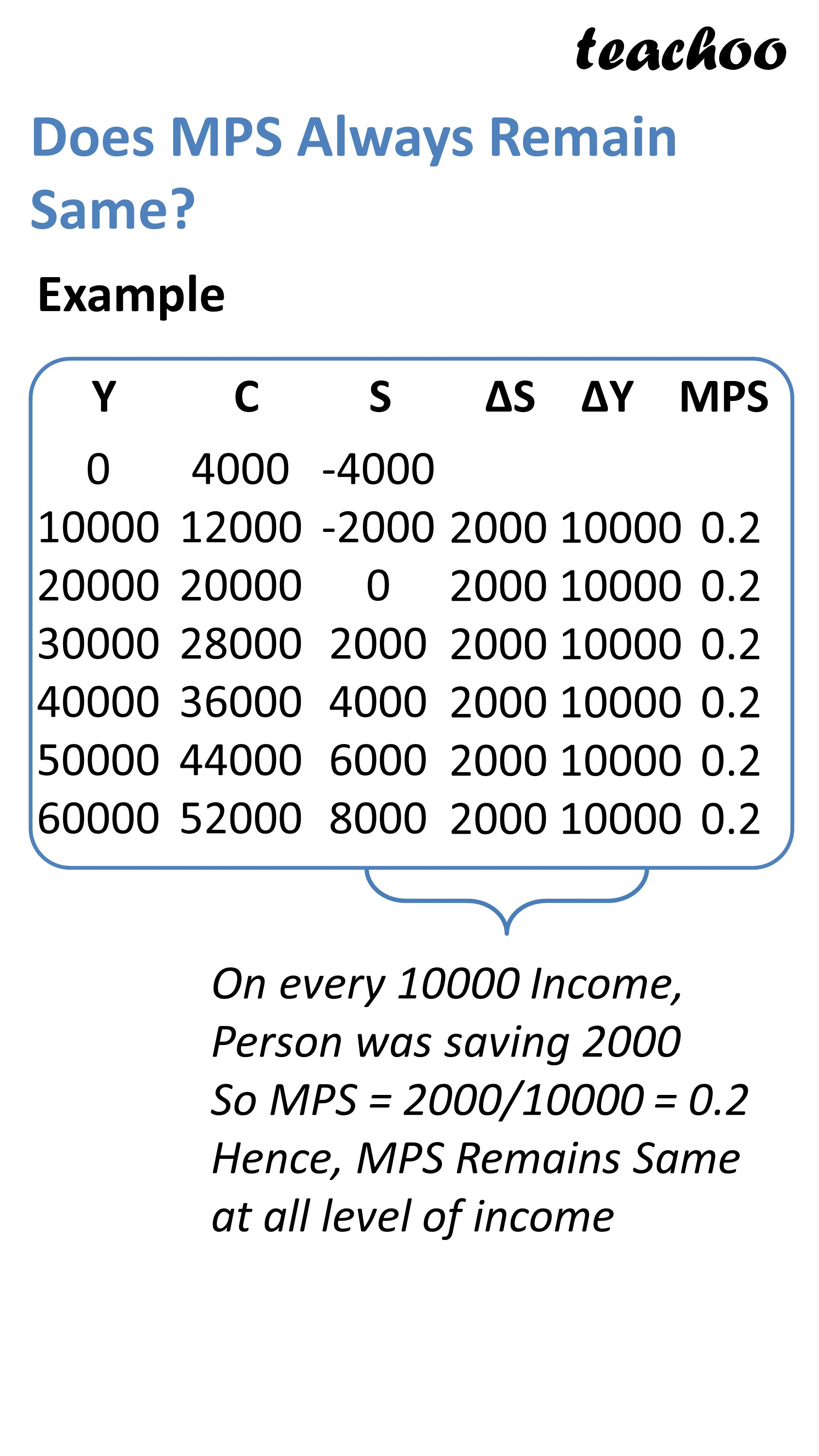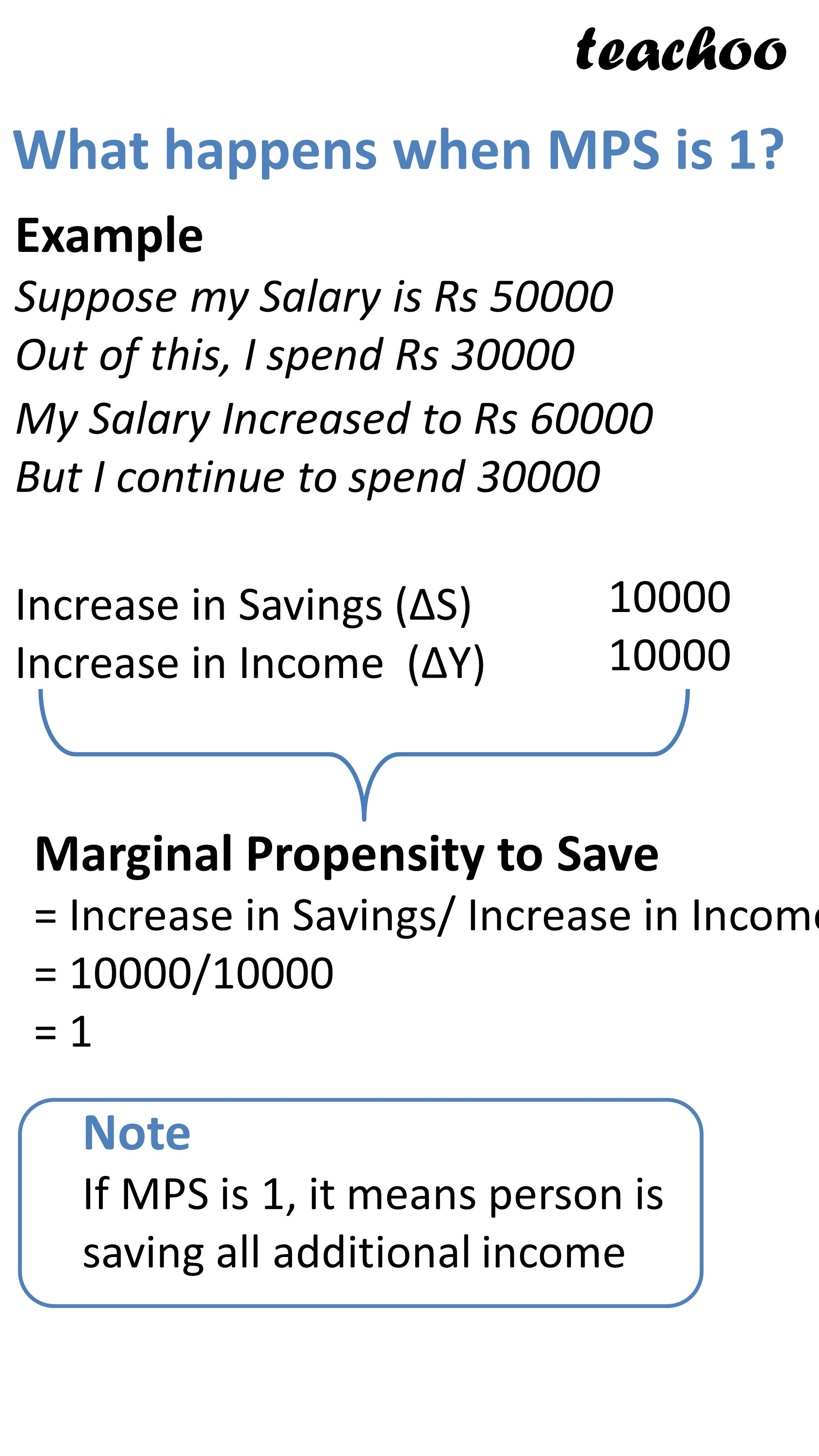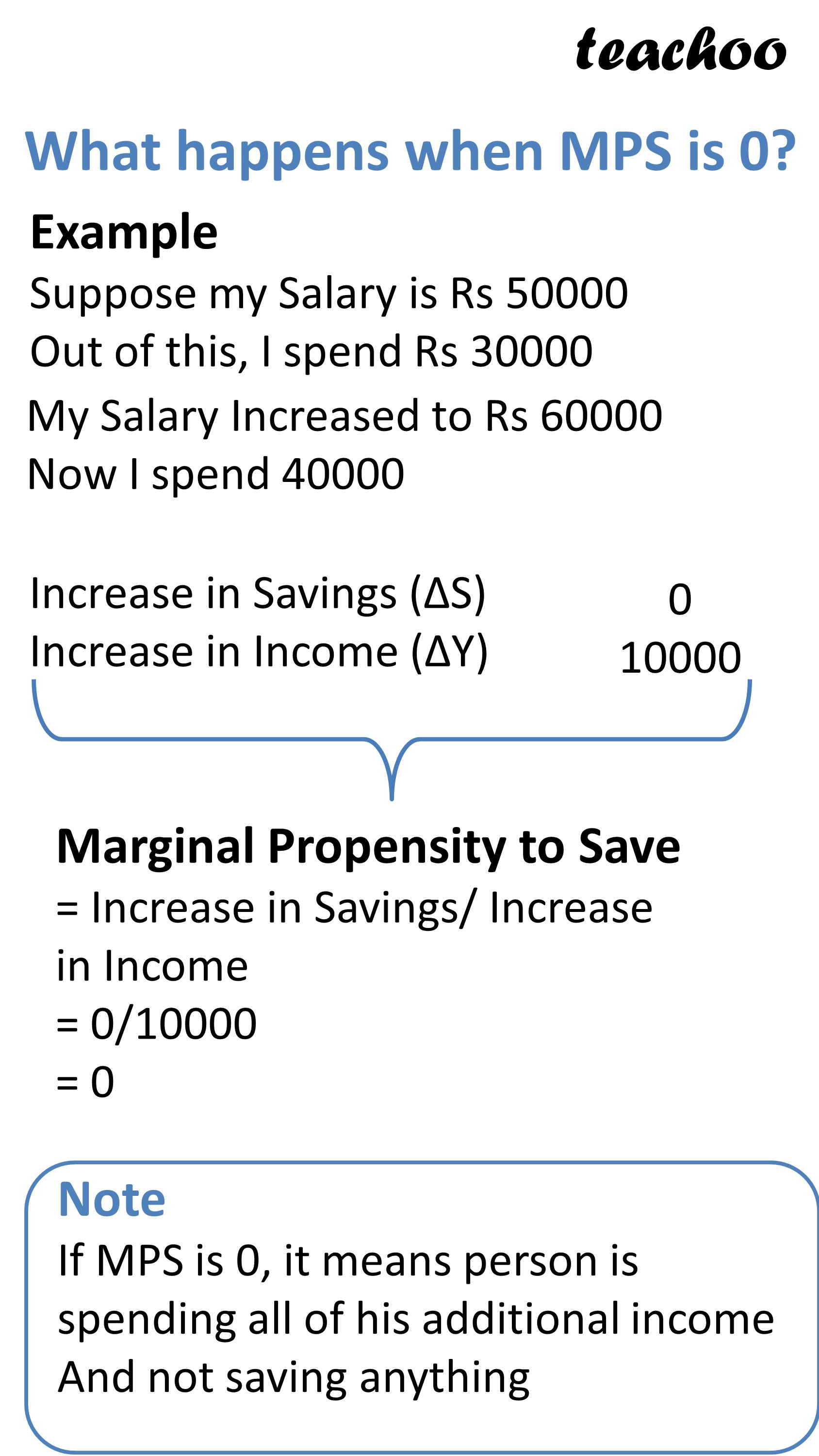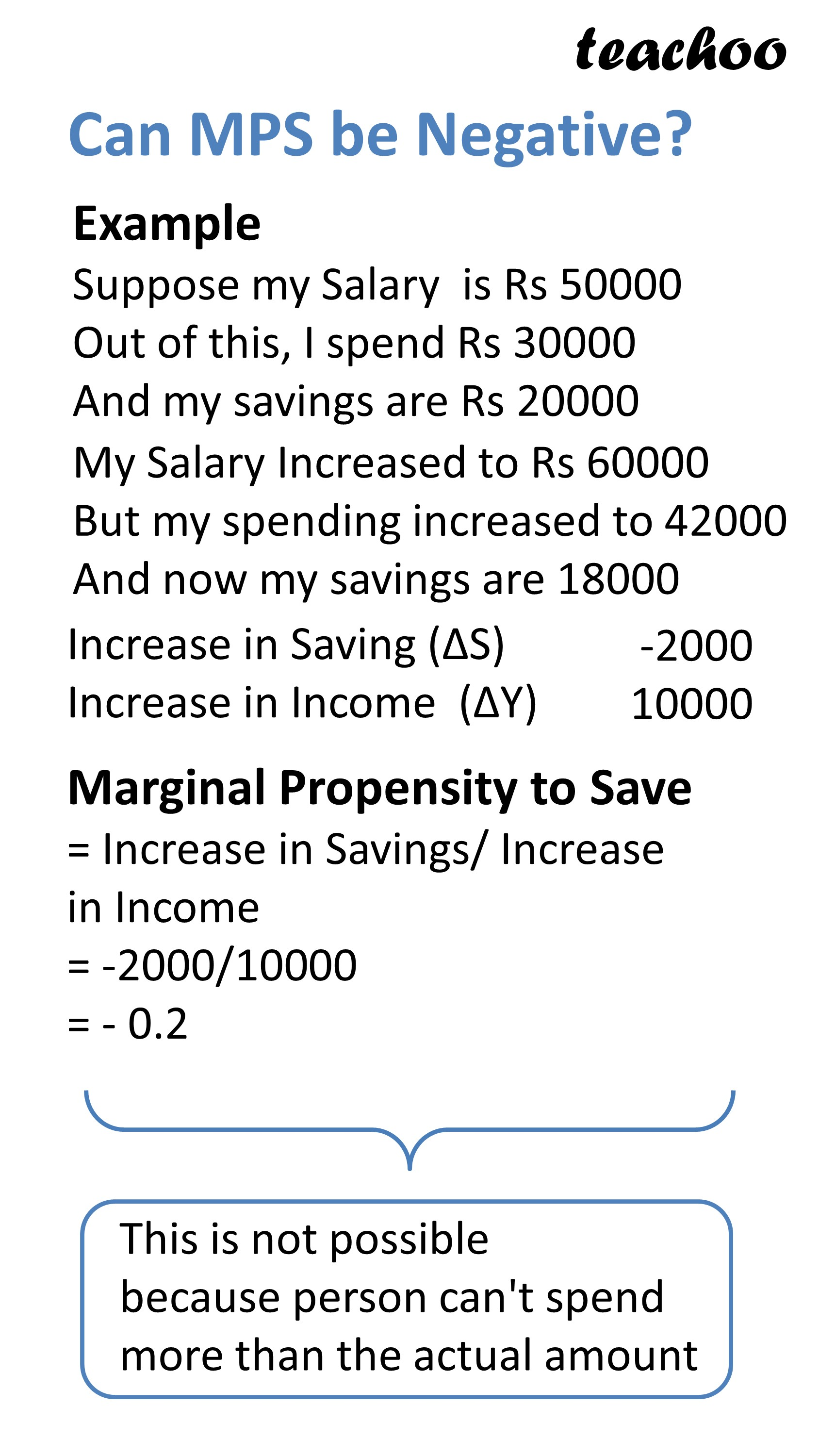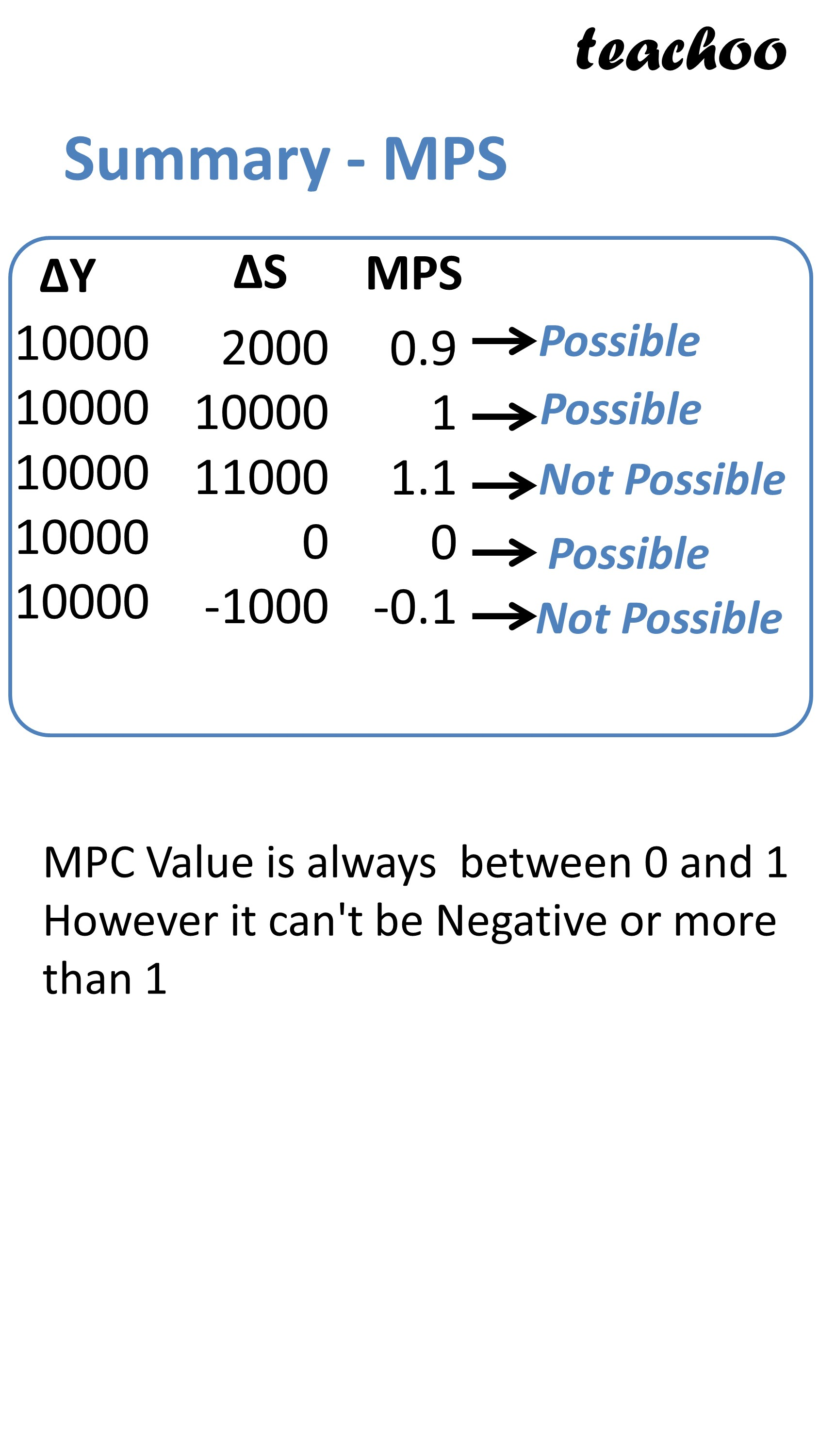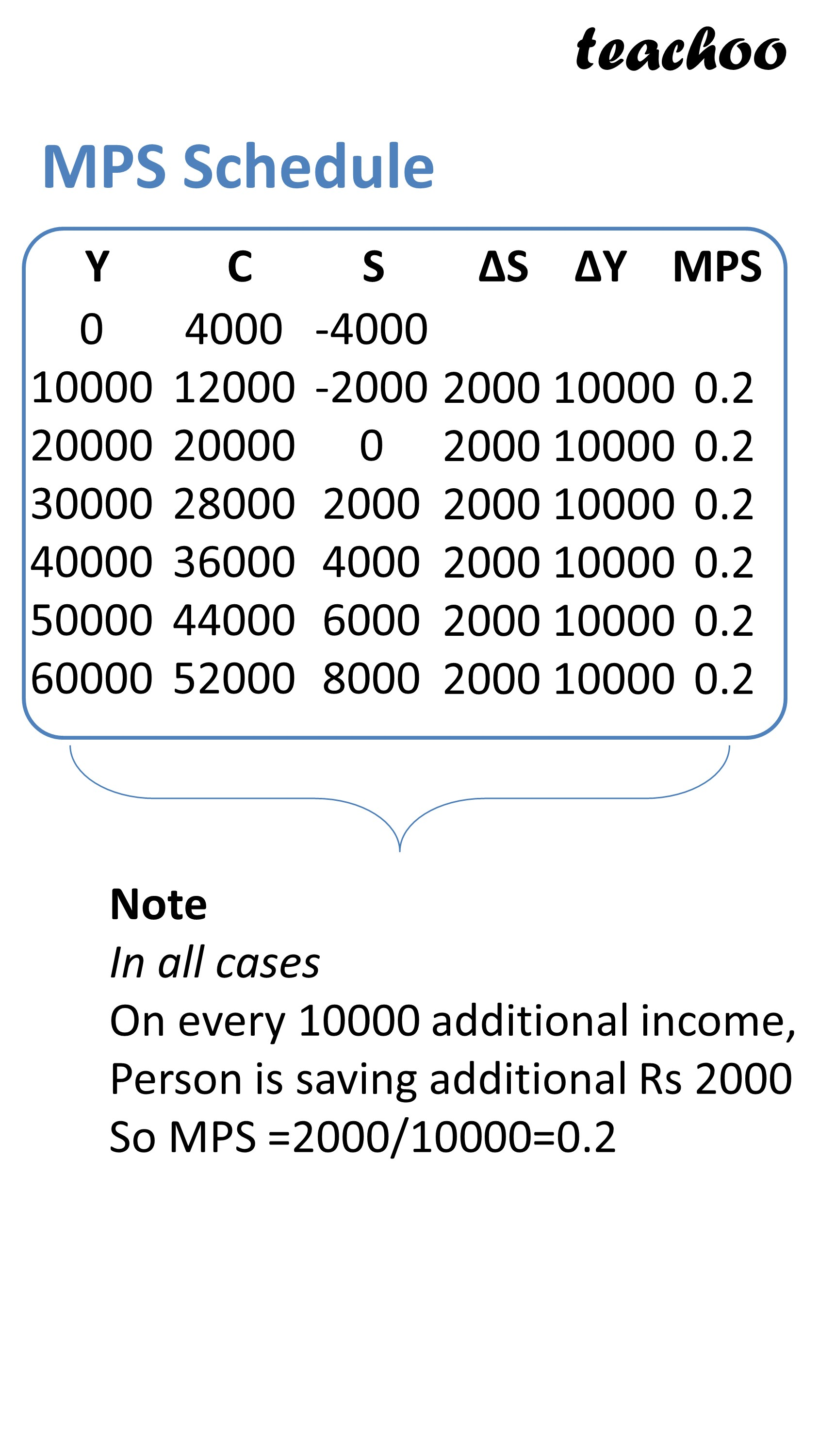Value of MPS is normally between 0 and 1

It remains same irrespective of level of income

## What happens when MPS is 0?

If MPC is 0, it means person is not saving additional income

Example

A person earned income of 40000 out of which he spent 30000 on consumption and saving 10000

A person's income increases from 40000 to 50000

However his consumption also increased to 40000 and saving remains 10000

Calculate MPS

Ans

Change in Savings = 0

Change in Income = 50000 - 40000 = 10000

MPS = Change in Consumption/ Change in Income

= 0/10000

= 0

## What happens when MPS = 1?

It means person is saving all of his additional income

Example 1

A person earned income of 40000 out of which he spent 30000 on consumption and saving 10000

A person's income increases from 40000 to 50000

However his consumption remained 30000 and saving became 20000

Change in Savings = 20000 - 10000 = 10000

Change in Income = 50000 - 40000 = 10000

MPS = Change in Savings/Change in Income

= 10000/10000

= 1

## Why can't MPS be more than 1?

If it is more than 1, it means person is saving more than his additional income

It can happen only if person's consumption exp decrease

Example

Person earns 10000 extra income but he saves 11000 extra

MPS = Change in Savings/Change in Income = 11000/ 10000 = 1.1

This is not possible

## How to show MPS in Graph

On X Axis, We represent Income

On Y Axis, We Represent Savings

It can be seen that

Savings Curve is Positively Sloping

However, it stats at Point S which is below the origin

Taking any 2 points on Savings curve, we can calculate MPS

We take points A and B

At Point A, Savings Level is P and Income level is Y2

At Point B, Savings Level is R and Income level is Y2

MPS = Change in Consumption/Change in Income

= PR/ Y1Y2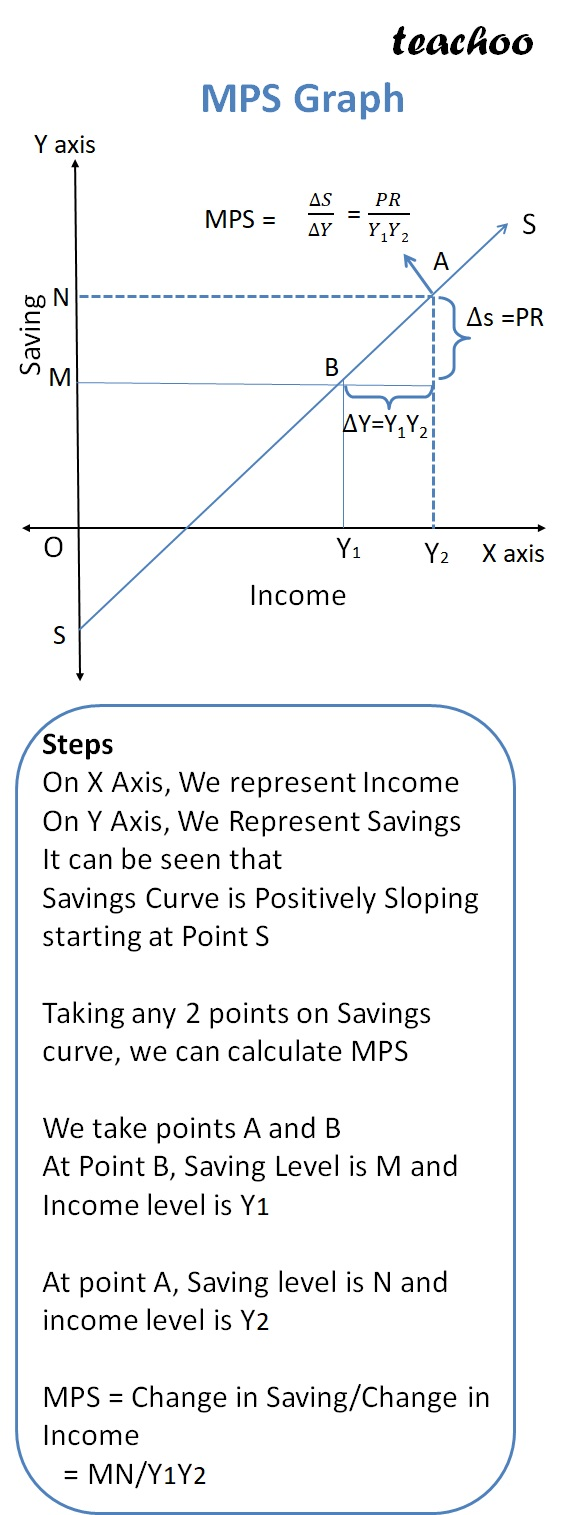### NCERT Questions

No questions in this part

### Other Books

#### Question 1

State the difference between APS and MPS.

Get live Maths 1-on-1 Classs - Class 6 to 12

### Transcript

What is Marginal Propensity to Save (MPS) Example 1 Suppose my Salary is Rs 50000 Out of this, I spend Rs 30000 and save 20000 My Salary Increased to Rs 60000 I now spend Rs 36000 and save 24000 Change in Saving (ΔS) = Change in Income (ΔY) = Ratio of these 2 is MPS MPS=ΔS/ ΔY Marginal Propensity to Save = Change in Saving/Change in Income = ΔS/ΔY = 4000/10000 = 0.4 Does MPS Always Remain Same? Example 0 10000 20000 30000 40000 50000 60000 4000 12000 20000 28000 36000 44000 52000 -4000 -2000 0 2000 4000 6000 8000 2000 2000 2000 2000 2000 2000 10000 10000 10000 10000 10000 10000 0.2 0.2 0.2 0.2 0.2 0.2 On every 10000 Income, Person was saving 2000 So MPS = 2000/10000 = 0.2 Hence, MPS Remains Same at all level of income What happens when MPS is 1? Example Suppose my Salary is Rs 50000 Out of this, I spend Rs 30000 My Salary Increased to Rs 60000 But I continue to spend 30000 Increase in Savings (ΔS) Increase in Income (ΔY) 10000 10000 Marginal Propensity to Save = Increase in Savings/ Increase in Income = 10000/10000 = 1 Note If MPS is 1, it means person is saving all additional income What happens when MPS is 0? Example Suppose my Salary is Rs 50000 Out of this, I spend Rs 30000 My Salary Increased to Rs 60000 Now I spend 40000 Increase in Savings (ΔS) Increase in Income (ΔY) Marginal Propensity to Save = Increase in Savings/ Increase in Income = 0/10000 = 0 Note If MPS is 0, it means person is spending all of his additional income And not saving anything Can MPS be more than 1? Example Suppose my Salary is Rs 50000 Out of this, I spend Rs 30000 My Salary Increased to Rs 60000 Now I spend 28000 Increase in Savings (ΔS) Increase in Income (ΔY) 12000 10000 Marginal Propensity to Save = Increase in Savings/ Increase in Income = 12000/10000 = 1.2 This is not possible because person can't save more than additional Income Can MPS be Negative? Example Suppose my Salary is Rs 50000 Out of this, I spend Rs 30000 My Salary Increased to Rs 60000 But my spending decreased to 32000 Increase in Saving (ΔS) Increase in Income (ΔY) -2000 10000 Marginal Propensity to Save = Increase in Savings/ Increase in Income = -2000/10000 = - 0.2 This is not possible because person can't spend more than the actual amount Summary - MPS 10000 10000 10000 10000 10000 2000 10000 11000 0 -1000 0.9 1 1.1 0 -0.1 MPC Value is always between 0 and 1 However it can't be Negative or more than 1 MPS Schedule 0 10000 20000 30000 40000 50000 60000 4000 12000 20000 28000 36000 44000 52000 -4000 -2000 0 2000 4000 6000 8000 2000 2000 2000 2000 2000 2000 10000 10000 10000 10000 10000 10000 0.2 0.2 0.2 0.2 0.2 0.2 MPS Graph Steps On X Axis, We represent Income On Y Axis, We Represent Savings It can be seen that Savings Curve is Positively Sloping starting at Point S Taking any 2 points on Savings curve, we can calculate MPS We take points A and B At Point B, Saving Level is M and Income level is Y1 At point A, Saving level is N and income level is Y2 MPS = Change in Saving/Change in Income = MN/Y1Y2